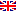Scheda programma d'esame
CALCOLO SCIENTIFICO
LIDIA ACETO
CdSMATEMATICA
Codice044AA
CFU6
PeriodoPrimo semestre
LinguaItaliano

ModuliSettore/iTipoOreDocente/i
CALCOLO SCIENTIFICOMAT/08LEZIONI60
 LIDIA ACETO unimap DARIO ANDREA BINI unimapEsporta in pdf
Programma non disponibile nella lingua selezionata
Learning outcomes
Knowledge

The student who successfully completes the course will be acquainted with numerical methods for solving linear algebra problems including advanced methods for linear systems and matrix eigenvalue computation. The student will also be aware of the modelling and the numerical treatment of several problems arising from some real-world applications of science, technology, math, and engineering.

Assessment criteria of knowledge

The written exam consists of the implementation and the experimental evaluation of a numerical algorithm. With the oral presentation the student must demonstrate the ability to approach a research article and organise an effective exposition of the results. Alternatively, during the oral exam the student must be able to demonstrate his/her knowledge of the course material.

Methods:

• Final oral exam
• Final written exam
Skills

At the end of the course students will have capability of analyzing numerical problems from the computational point of view and to provide Octave / Matlab implementation of numerical algorithms.

Assessment criteria of skills

The assessment criteria of skills rely on the implementation of  Matlab / Octave functions concerning specific problems and on the ability of students to demonstrate their knowledge of the main course contents using the appropriate terminology.

Behaviors

The students will reach a numerical thought where the concepts of numerical stability and conditioning play a fundamental role, and where minimizing the computational complexity is a continuous demand in the design and analysis of numerical algorithms.

Assessment criteria of behaviors

The implementation of  Matlab / Octave functions concerning specific problems and the ability of students to demonstrate his/her knowledge of the main course contents using the appropriate terminology are once again the main criteria for the assessment of behaviors.

Prerequisites

Basic notions of Linear Algebra (both theoretical and numerical) and of Calculus.

Teaching methods

Delivery: face to face

Learning activities:

• attending lectures
• participation in discussions
• individual study
• Laboratory work
• Practical

Attendance: Not mandatory

Teaching methods:

• Lectures
• laboratory
• project work
Syllabus

Numerical methods for eigenvalue computation. Numerical methods for SVD computation. Numerical methods for least squares problems. Iterative numerical methods for linear systems.

Bibliography